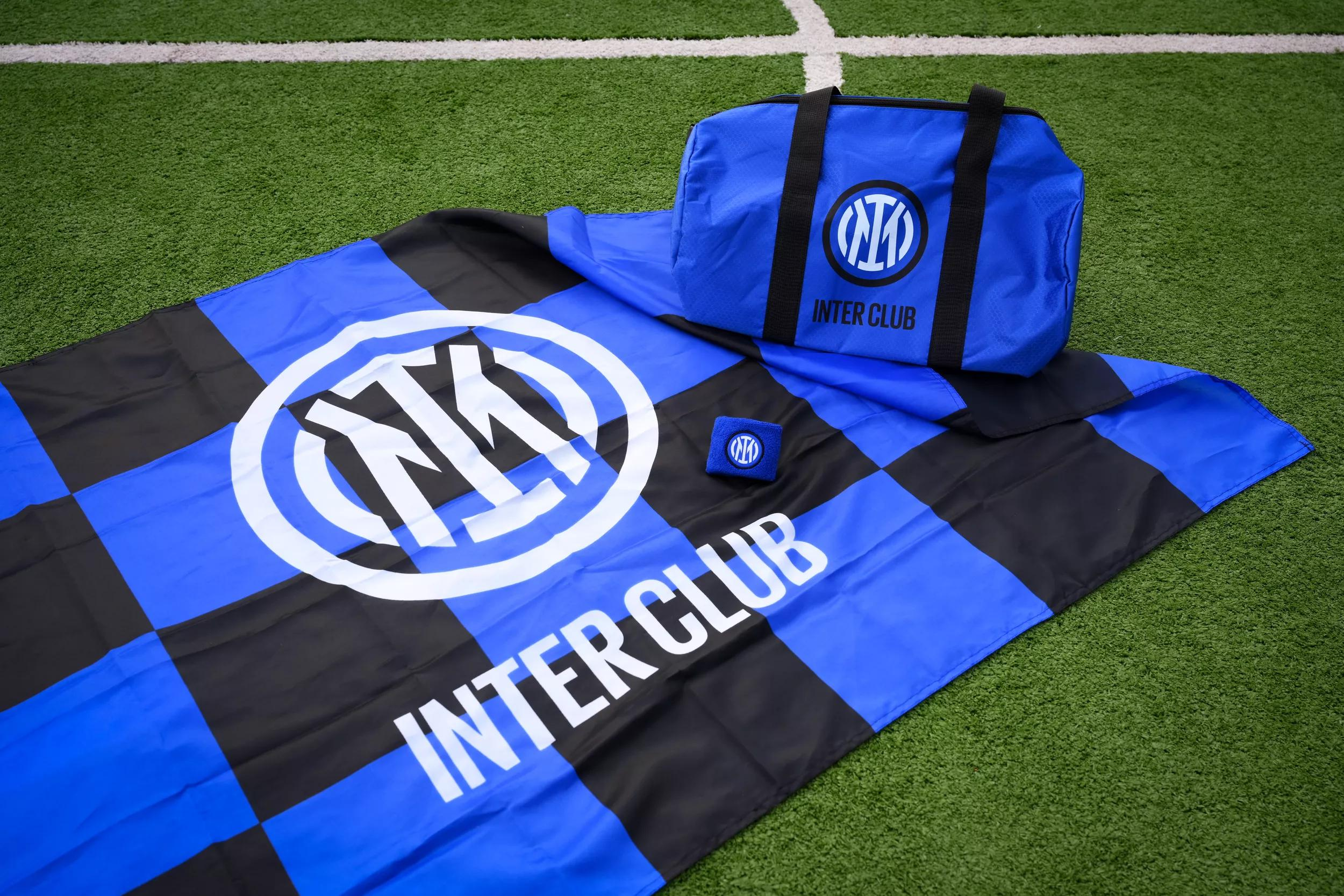# .css-adzqt3{display:inline-block;word-break:break-word;opacity:0;position:relative;-webkit-animation:animation-plwpox 0ms 0.4s forwards;animation:animation-plwpox 0ms 0.4s forwards;}.css-adzqt3::after{content:"";position:absolute;width:calc(100% + 14px);height:100%;top:0px;left:0px;background-color:var(--chakra-colors-light-white_100);-webkit-transform:scale3d(0, 1.05, 1);-moz-transform:scale3d(0, 1.05, 1);-ms-transform:scale3d(0, 1.05, 1);transform:scale3d(0, 1.05, 1);-webkit-animation:animation-f981je 250ms 0.15000000000000002s forwards step-end,animation-1ncn112 250ms 0.65s forwards step-end;animation:animation-f981je 250ms 0.15000000000000002s forwards step-end,animation-1ncn112 250ms 0.65s forwards step-end;}@-webkit-keyframes animation-1ncn112{0%{-webkit-transform:scale3d(0.75, 1.05, 1);-moz-transform:scale3d(0.75, 1.05, 1);-ms-transform:scale3d(0.75, 1.05, 1);transform:scale3d(0.75, 1.05, 1);transform-origin:top right;}44.4%{-webkit-transform:scale3d(0.25, 1.05, 1);-moz-transform:scale3d(0.25, 1.05, 1);-ms-transform:scale3d(0.25, 1.05, 1);transform:scale3d(0.25, 1.05, 1);transform-origin:top right;}100%{-webkit-transform:scale3d(0, 1.05, 1);-moz-transform:scale3d(0, 1.05, 1);-ms-transform:scale3d(0, 1.05, 1);transform:scale3d(0, 1.05, 1);transform-origin:top right;}}@keyframes animation-1ncn112{0%{-webkit-transform:scale3d(0.75, 1.05, 1);-moz-transform:scale3d(0.75, 1.05, 1);-ms-transform:scale3d(0.75, 1.05, 1);transform:scale3d(0.75, 1.05, 1);transform-origin:top right;}44.4%{-webkit-transform:scale3d(0.25, 1.05, 1);-moz-transform:scale3d(0.25, 1.05, 1);-ms-transform:scale3d(0.25, 1.05, 1);transform:scale3d(0.25, 1.05, 1);transform-origin:top right;}100%{-webkit-transform:scale3d(0, 1.05, 1);-moz-transform:scale3d(0, 1.05, 1);-ms-transform:scale3d(0, 1.05, 1);transform:scale3d(0, 1.05, 1);transform-origin:top right;}}@-webkit-keyframes animation-f981je{0%{-webkit-transform:scale3d(0, 1.05, 1);-moz-transform:scale3d(0, 1.05, 1);-ms-transform:scale3d(0, 1.05, 1);transform:scale3d(0, 1.05, 1);transform-origin:top left;}22.2%{-webkit-transform:scale3d(0.25, 1.05, 1);-moz-transform:scale3d(0.25, 1.05, 1);-ms-transform:scale3d(0.25, 1.05, 1);transform:scale3d(0.25, 1.05, 1);transform-origin:top left;}44.4%{-webkit-transform:scale3d(0.5, 1.05, 1);-moz-transform:scale3d(0.5, 1.05, 1);-ms-transform:scale3d(0.5, 1.05, 1);transform:scale3d(0.5, 1.05, 1);transform-origin:top left;}66.6%{-webkit-transform:scale3d(0.75, 1.05, 1);-moz-transform:scale3d(0.75, 1.05, 1);-ms-transform:scale3d(0.75, 1.05, 1);transform:scale3d(0.75, 1.05, 1);transform-origin:top left;}100%{-webkit-transform:scale3d(1, 1.05, 1);-moz-transform:scale3d(1, 1.05, 1);-ms-transform:scale3d(1, 1.05, 1);transform:scale3d(1, 1.05, 1);transform-origin:top left;}}@keyframes animation-f981je{0%{-webkit-transform:scale3d(0, 1.05, 1);-moz-transform:scale3d(0, 1.05, 1);-ms-transform:scale3d(0, 1.05, 1);transform:scale3d(0, 1.05, 1);transform-origin:top left;}22.2%{-webkit-transform:scale3d(0.25, 1.05, 1);-moz-transform:scale3d(0.25, 1.05, 1);-ms-transform:scale3d(0.25, 1.05, 1);transform:scale3d(0.25, 1.05, 1);transform-origin:top left;}44.4%{-webkit-transform:scale3d(0.5, 1.05, 1);-moz-transform:scale3d(0.5, 1.05, 1);-ms-transform:scale3d(0.5, 1.05, 1);transform:scale3d(0.5, 1.05, 1);transform-origin:top left;}66.6%{-webkit-transform:scale3d(0.75, 1.05, 1);-moz-transform:scale3d(0.75, 1.05, 1);-ms-transform:scale3d(0.75, 1.05, 1);transform:scale3d(0.75, 1.05, 1);transform-origin:top left;}100%{-webkit-transform:scale3d(1, 1.05, 1);-moz-transform:scale3d(1, 1.05, 1);-ms-transform:scale3d(1, 1.05, 1);transform:scale3d(1, 1.05, 1);transform-origin:top left;}}@-webkit-keyframes animation-plwpox{from{opacity:0;}to{opacity:1;}}@keyframes animation-plwpox{from{opacity:0;}to{opacity:1;}}INTER.css-z4mzdx{display:inline-block;word-break:break-word;opacity:0;position:relative;-webkit-animation:animation-plwpox 0ms 0.65s forwards;animation:animation-plwpox 0ms 0.65s forwards;}.css-z4mzdx::after{content:"";position:absolute;width:calc(100% + 14px);height:100%;top:0px;left:0px;background-color:var(--chakra-colors-light-white_100);-webkit-transform:scale3d(0, 1.05, 1);-moz-transform:scale3d(0, 1.05, 1);-ms-transform:scale3d(0, 1.05, 1);transform:scale3d(0, 1.05, 1);-webkit-animation:animation-f981je 250ms 0.4s forwards step-end,animation-1ncn112 250ms 0.9s forwards step-end;animation:animation-f981je 250ms 0.4s forwards step-end,animation-1ncn112 250ms 0.9s forwards step-end;}@-webkit-keyframes animation-1ncn112{0%{-webkit-transform:scale3d(0.75, 1.05, 1);-moz-transform:scale3d(0.75, 1.05, 1);-ms-transform:scale3d(0.75, 1.05, 1);transform:scale3d(0.75, 1.05, 1);transform-origin:top right;}44.4%{-webkit-transform:scale3d(0.25, 1.05, 1);-moz-transform:scale3d(0.25, 1.05, 1);-ms-transform:scale3d(0.25, 1.05, 1);transform:scale3d(0.25, 1.05, 1);transform-origin:top right;}100%{-webkit-transform:scale3d(0, 1.05, 1);-moz-transform:scale3d(0, 1.05, 1);-ms-transform:scale3d(0, 1.05, 1);transform:scale3d(0, 1.05, 1);transform-origin:top right;}}@keyframes animation-1ncn112{0%{-webkit-transform:scale3d(0.75, 1.05, 1);-moz-transform:scale3d(0.75, 1.05, 1);-ms-transform:scale3d(0.75, 1.05, 1);transform:scale3d(0.75, 1.05, 1);transform-origin:top right;}44.4%{-webkit-transform:scale3d(0.25, 1.05, 1);-moz-transform:scale3d(0.25, 1.05, 1);-ms-transform:scale3d(0.25, 1.05, 1);transform:scale3d(0.25, 1.05, 1);transform-origin:top right;}100%{-webkit-transform:scale3d(0, 1.05, 1);-moz-transform:scale3d(0, 1.05, 1);-ms-transform:scale3d(0, 1.05, 1);transform:scale3d(0, 1.05, 1);transform-origin:top right;}}@-webkit-keyframes animation-f981je{0%{-webkit-transform:scale3d(0, 1.05, 1);-moz-transform:scale3d(0, 1.05, 1);-ms-transform:scale3d(0, 1.05, 1);transform:scale3d(0, 1.05, 1);transform-origin:top left;}22.2%{-webkit-transform:scale3d(0.25, 1.05, 1);-moz-transform:scale3d(0.25, 1.05, 1);-ms-transform:scale3d(0.25, 1.05, 1);transform:scale3d(0.25, 1.05, 1);transform-origin:top left;}44.4%{-webkit-transform:scale3d(0.5, 1.05, 1);-moz-transform:scale3d(0.5, 1.05, 1);-ms-transform:scale3d(0.5, 1.05, 1);transform:scale3d(0.5, 1.05, 1);transform-origin:top left;}66.6%{-webkit-transform:scale3d(0.75, 1.05, 1);-moz-transform:scale3d(0.75, 1.05, 1);-ms-transform:scale3d(0.75, 1.05, 1);transform:scale3d(0.75, 1.05, 1);transform-origin:top left;}100%{-webkit-transform:scale3d(1, 1.05, 1);-moz-transform:scale3d(1, 1.05, 1);-ms-transform:scale3d(1, 1.05, 1);transform:scale3d(1, 1.05, 1);transform-origin:top left;}}@keyframes animation-f981je{0%{-webkit-transform:scale3d(0, 1.05, 1);-moz-transform:scale3d(0, 1.05, 1);-ms-transform:scale3d(0, 1.05, 1);transform:scale3d(0, 1.05, 1);transform-origin:top left;}22.2%{-webkit-transform:scale3d(0.25, 1.05, 1);-moz-transform:scale3d(0.25, 1.05, 1);-ms-transform:scale3d(0.25, 1.05, 1);transform:scale3d(0.25, 1.05, 1);transform-origin:top left;}44.4%{-webkit-transform:scale3d(0.5, 1.05, 1);-moz-transform:scale3d(0.5, 1.05, 1);-ms-transform:scale3d(0.5, 1.05, 1);transform:scale3d(0.5, 1.05, 1);transform-origin:top left;}66.6%{-webkit-transform:scale3d(0.75, 1.05, 1);-moz-transform:scale3d(0.75, 1.05, 1);-ms-transform:scale3d(0.75, 1.05, 1);transform:scale3d(0.75, 1.05, 1);transform-origin:top left;}100%{-webkit-transform:scale3d(1, 1.05, 1);-moz-transform:scale3d(1, 1.05, 1);-ms-transform:scale3d(1, 1.05, 1);transform:scale3d(1, 1.05, 1);transform-origin:top left;}}@-webkit-keyframes animation-plwpox{from{opacity:0;}to{opacity:1;}}@keyframes animation-plwpox{from{opacity:0;}to{opacity:1;}}CLUB.css-1hstmlz{display:inline-block;word-break:break-word;opacity:0;position:relative;-webkit-animation:animation-plwpox 0ms 0.9s forwards;animation:animation-plwpox 0ms 0.9s forwards;}.css-1hstmlz::after{content:"";position:absolute;width:calc(100% + 14px);height:100%;top:0px;left:0px;background-color:var(--chakra-colors-light-white_100);-webkit-transform:scale3d(0, 1.05, 1);-moz-transform:scale3d(0, 1.05, 1);-ms-transform:scale3d(0, 1.05, 1);transform:scale3d(0, 1.05, 1);-webkit-animation:animation-f981je 250ms 0.65s forwards step-end,animation-1ncn112 250ms 1.15s forwards step-end;animation:animation-f981je 250ms 0.65s forwards step-end,animation-1ncn112 250ms 1.15s forwards step-end;}@-webkit-keyframes animation-1ncn112{0%{-webkit-transform:scale3d(0.75, 1.05, 1);-moz-transform:scale3d(0.75, 1.05, 1);-ms-transform:scale3d(0.75, 1.05, 1);transform:scale3d(0.75, 1.05, 1);transform-origin:top right;}44.4%{-webkit-transform:scale3d(0.25, 1.05, 1);-moz-transform:scale3d(0.25, 1.05, 1);-ms-transform:scale3d(0.25, 1.05, 1);transform:scale3d(0.25, 1.05, 1);transform-origin:top right;}100%{-webkit-transform:scale3d(0, 1.05, 1);-moz-transform:scale3d(0, 1.05, 1);-ms-transform:scale3d(0, 1.05, 1);transform:scale3d(0, 1.05, 1);transform-origin:top right;}}@keyframes animation-1ncn112{0%{-webkit-transform:scale3d(0.75, 1.05, 1);-moz-transform:scale3d(0.75, 1.05, 1);-ms-transform:scale3d(0.75, 1.05, 1);transform:scale3d(0.75, 1.05, 1);transform-origin:top right;}44.4%{-webkit-transform:scale3d(0.25, 1.05, 1);-moz-transform:scale3d(0.25, 1.05, 1);-ms-transform:scale3d(0.25, 1.05, 1);transform:scale3d(0.25, 1.05, 1);transform-origin:top right;}100%{-webkit-transform:scale3d(0, 1.05, 1);-moz-transform:scale3d(0, 1.05, 1);-ms-transform:scale3d(0, 1.05, 1);transform:scale3d(0, 1.05, 1);transform-origin:top right;}}@-webkit-keyframes animation-f981je{0%{-webkit-transform:scale3d(0, 1.05, 1);-moz-transform:scale3d(0, 1.05, 1);-ms-transform:scale3d(0, 1.05, 1);transform:scale3d(0, 1.05, 1);transform-origin:top left;}22.2%{-webkit-transform:scale3d(0.25, 1.05, 1);-moz-transform:scale3d(0.25, 1.05, 1);-ms-transform:scale3d(0.25, 1.05, 1);transform:scale3d(0.25, 1.05, 1);transform-origin:top left;}44.4%{-webkit-transform:scale3d(0.5, 1.05, 1);-moz-transform:scale3d(0.5, 1.05, 1);-ms-transform:scale3d(0.5, 1.05, 1);transform:scale3d(0.5, 1.05, 1);transform-origin:top left;}66.6%{-webkit-transform:scale3d(0.75, 1.05, 1);-moz-transform:scale3d(0.75, 1.05, 1);-ms-transform:scale3d(0.75, 1.05, 1);transform:scale3d(0.75, 1.05, 1);transform-origin:top left;}100%{-webkit-transform:scale3d(1, 1.05, 1);-moz-transform:scale3d(1, 1.05, 1);-ms-transform:scale3d(1, 1.05, 1);transform:scale3d(1, 1.05, 1);transform-origin:top left;}}@keyframes animation-f981je{0%{-webkit-transform:scale3d(0, 1.05, 1);-moz-transform:scale3d(0, 1.05, 1);-ms-transform:scale3d(0, 1.05, 1);transform:scale3d(0, 1.05, 1);transform-origin:top left;}22.2%{-webkit-transform:scale3d(0.25, 1.05, 1);-moz-transform:scale3d(0.25, 1.05, 1);-ms-transform:scale3d(0.25, 1.05, 1);transform:scale3d(0.25, 1.05, 1);transform-origin:top left;}44.4%{-webkit-transform:scale3d(0.5, 1.05, 1);-moz-transform:scale3d(0.5, 1.05, 1);-ms-transform:scale3d(0.5, 1.05, 1);transform:scale3d(0.5, 1.05, 1);transform-origin:top left;}66.6%{-webkit-transform:scale3d(0.75, 1.05, 1);-moz-transform:scale3d(0.75, 1.05, 1);-ms-transform:scale3d(0.75, 1.05, 1);transform:scale3d(0.75, 1.05, 1);transform-origin:top left;}100%{-webkit-transform:scale3d(1, 1.05, 1);-moz-transform:scale3d(1, 1.05, 1);-ms-transform:scale3d(1, 1.05, 1);transform:scale3d(1, 1.05, 1);transform-origin:top left;}}@-webkit-keyframes animation-plwpox{from{opacity:0;}to{opacity:1;}}@keyframes animation-plwpox{from{opacity:0;}to{opacity:1;}}

###### For over 60 years, Inter Clubs have waved the Nerazzurri colours around the world with pride and passion! Become part of the international Inter Club community, JOIN THE FAMILY!

SEASON 2023/2024

SOLD OUT

WELCOME KIT## SERVICES AND BENEFITS### TICKETING AND DEDICATED ACCESS

Ticket sales phase dedicated to big matches and European away games, as well as a dedicated Inter Club Members entrance to the stadium### DISCOUNTS AND CONVENTIONS

Find out more about discounts on merchandising and all the conventions for Inter Club affiliates### ACTIVITIES AND EXPERIENCE

Find out more about the experiences and activities dedicated to Inter Club members